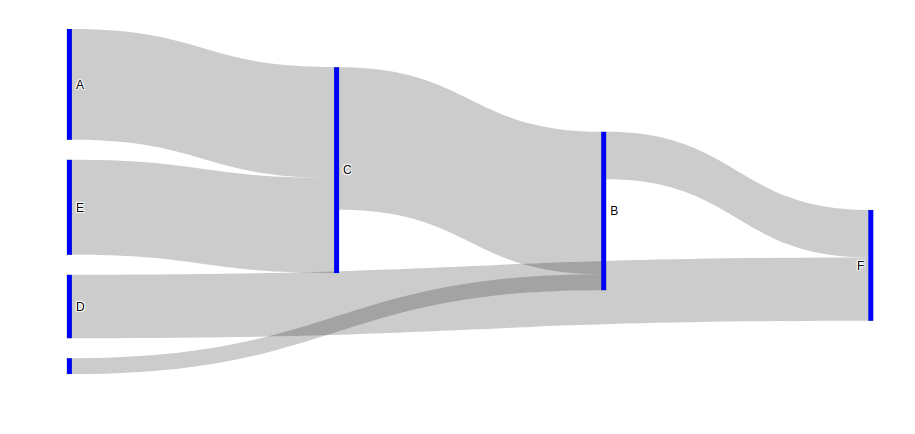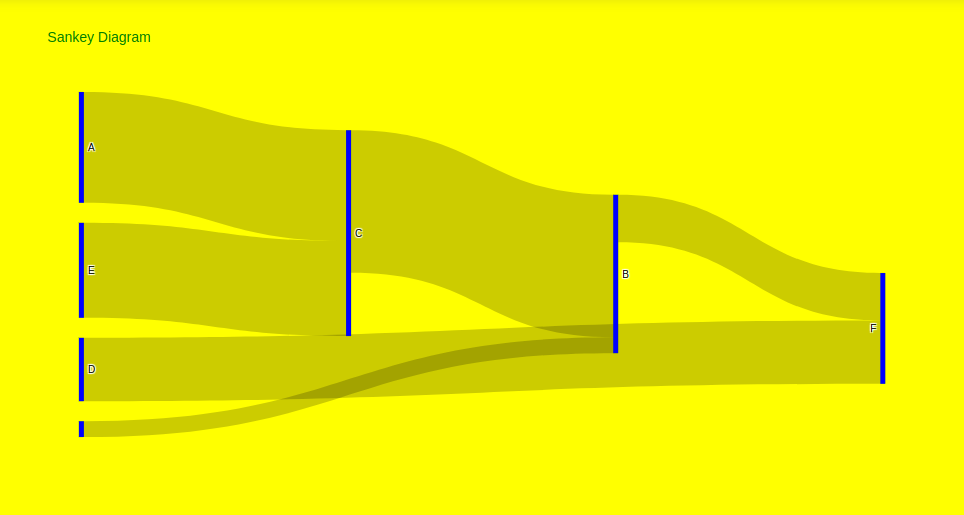# Sankey Diagram using Plotly in Python

• Last Updated : 05 Sep, 2020

Plotly is a Python library that is used to design graphs, especially interactive graphs. It can plot various graphs and charts like histogram, barplot, boxplot, spreadplot, and many more. It is mainly used in data analysis as well as financial analysis. plotly is an interactive visualization library.

## Sankey Diagrams

Sankey diagrams are a type of flow diagram in which the width of the arrows is comparative to the flow rate. Sankey diagrams can also visualize the source to represent the source node, target for the target node, value to set the flow volume and label that shows the node name. If the flow is twice as wide it represents double the quantity.

Example 1:

## Python3

 `fig ``=` `go.Figure(data``=``[go.Sankey(``    ``node ``=` `dict``(``      ``thickness ``=` `5``,``      ``line ``=` `dict``(color ``=` `"green"``, width ``=` `0.1``),``      ``label ``=` `[``"A"``, ``"B"``, ``"C"``, ``"D"``, ``"E"``, ``"F"``],``      ``color ``=` `"blue"``    ``),``    ``link ``=` `dict``(``         ` `      ``# indices correspond to labels``      ``source ``=` `[``0``, ``6``, ``1``, ``4``, ``2``, ``3``], ``      ``target ``=` `[``2``, ``1``, ``5``, ``2``, ``1``, ``5``],``      ``value ``=` `[``7``, ``1``, ``3``, ``6``, ``9``, ``4``]``  ``))])`` ` `fig.show()`

Output:Example 2: Styling the graph

## Python3

 `fig ``=` `go.Figure(data``=``[go.Sankey(``    ``node ``=` `dict``(``      ``thickness ``=` `5``,``      ``line ``=` `dict``(color ``=` `"green"``, width ``=` `0.1``),``      ``label ``=` `[``"A"``, ``"B"``, ``"C"``, ``"D"``, ``"E"``, ``"F"``],``      ``color ``=` `"blue"``    ``),``    ``link ``=` `dict``(``         ` `      ``# indices correspond to labels``      ``source ``=` `[``0``, ``6``, ``1``, ``4``, ``2``, ``3``], ``      ``target ``=` `[``2``, ``1``, ``5``, ``2``, ``1``, ``5``],``      ``value ``=` `[``7``, ``1``, ``3``, ``6``, ``9``, ``4``]``  ``))])`` ` `fig.update_layout(``    ``hovermode ``=` `'x'``,``    ``title``=``"Sankey Diagram"``,``    ``font``=``dict``(size ``=` `10``, color ``=` `'green'``),``    ``plot_bgcolor``=``'blue'``,``    ``paper_bgcolor``=``'yellow'``)`` ` `fig.show()`

Output:My Personal Notes arrow_drop_up